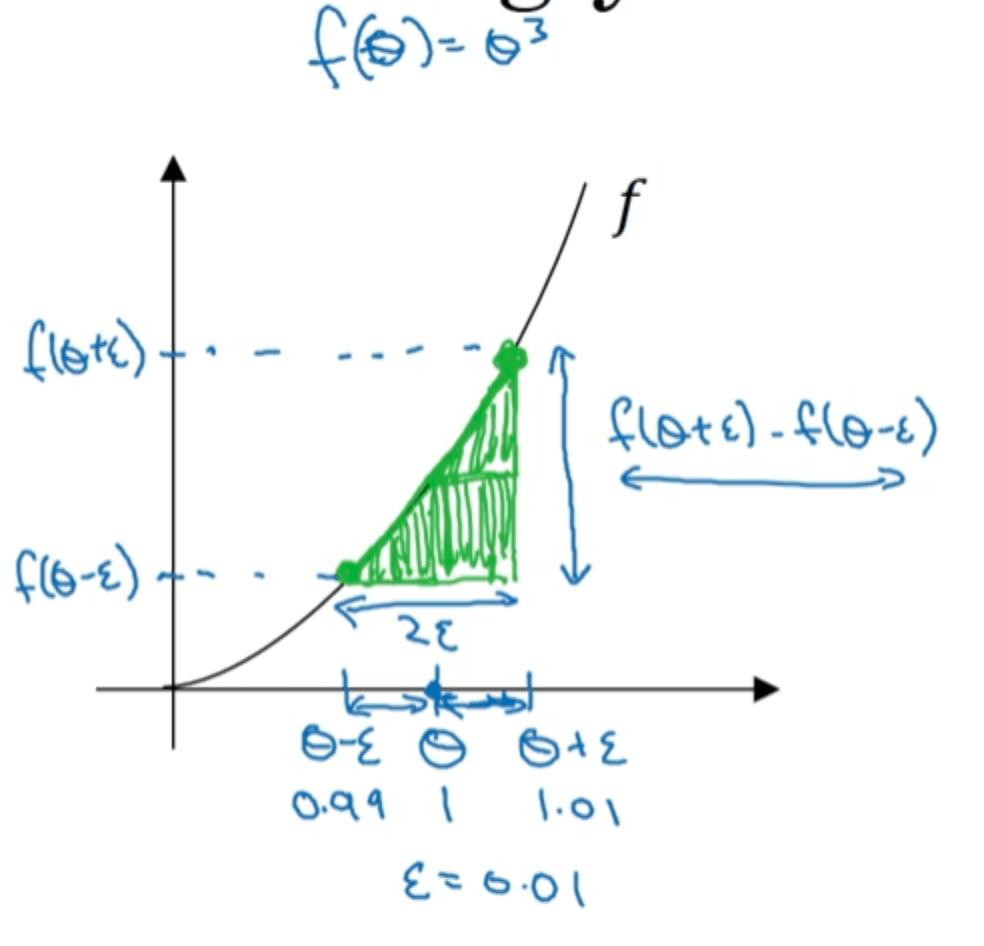# 【深度学习基础】第十四课：梯度检验

## 梯度的数值逼近，梯度检验

Posted by x-jeff on February 12, 2020

【深度学习基础】系列博客为学习Coursera上吴恩达深度学习课程所做的课程笔记。

# 2.梯度的数值逼近$\frac{f(\theta+\epsilon)-f(\theta)}{\epsilon}=\frac{1.01^3-1}{0.01}=3.0301$

$\frac{f(\theta+\epsilon)-f(\theta-\epsilon)}{2\epsilon}=\frac{1.01^3-0.99^3}{2\times 0.01}=3.0001$

$\theta=1$时，函数的导数为：

$f'(\theta)=3\theta ^2=3$

⚠️我们在做梯度检验时就会用到双边误差公式。

# 3.梯度检验

cost function为：

$J(W^{},b^{},...,W^{[L]},b^{[L]})=J(\theta_1,\theta_2,...)=J(\theta)$

$d\theta_{approx}[i]=\frac{J(\theta_1,\theta_2,...,\theta_i+\epsilon,...)-J(\theta_1,\theta_2,...,\theta_i-\epsilon,...)}{2\epsilon}$

$\frac{\parallel d\theta_{approx} - d\theta \parallel _2}{\parallel d\theta_{approx} \parallel _2+\parallel d\theta \parallel _2} \approx \epsilon$

# 4.梯度检验的实现技巧和注意事项

• 不要在训练中使用梯度检验，它只用于调试。因为梯度检验的过程会很慢。
• 如果算法的梯度检验失败，要检查每一项，试着找出bug。
• 在实施梯度检验时，如果使用了正则化，一定要记着在计算时带上正则项。
• 梯度检验不能与dropout同时使用。
• 当W和b接近0时，梯度检验可能总是正确的。但是W和b非常接近0的情况很少见。

# 5.代码地址